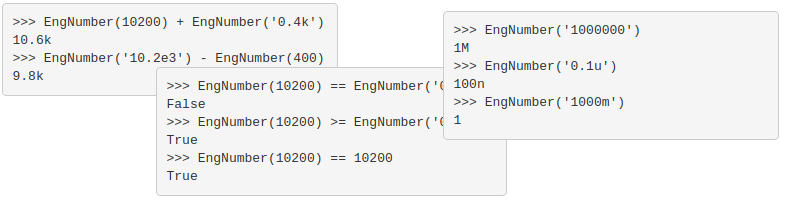# Engineering Notation

Anyone reading this will likely already have a background that covers engineering notation, so I won't go into it deeply. Computer scientists might also call engineering notation a 'human-readable' numbers. Instead of `4096B` you might see `4kB` or `4.096kB`, depending on your precision.

There are more expansive explanations all over the web if you want more information.

# Motivation

## Explanation of Decimal Class

The `Decimal` class is used when you want to do math the way that you learned to do it in school. Numbers are not represented in the processor as binary equivalents, they are represented as the exact value. Slower, but more precise.

The python `Decimal` class actually has a built-in method `to_eng_string()` appears to be written for this purpose, but actually doesn't work strictly for all numbers, meaning that:

```>>> Decimal('1000000').to_eng_string()
1000000
```

This is not engineering notation! But it is in line with the standard that is being implemented by `Decimal`. You have to actually give the string value in scientific notation OR call the `normalize` method:

```>>> Decimal('1000000').normalize().to_eng_string()
100E+3
```

Unfortunately, it doesn't work as well for fractional numbers:

```>>> Decimal('0.01').to_eng_string()
0.01
>>> Decimal('0.01').normalize().to_eng_string()
0.01
```

This behavior isn't a fault in the library. As some have explained, the library is doing exactly what it is written to do. It just isn't exactly what I want.

## What Do I Want?

Ideally, I want any number to always resolve into a human-readable form presented in every ENG 101 class:

```>>> EngNumber('1000000')
1M
>>> EngNumber('0.1u')
100n
>>> EngNumber('1000m')
1
```

To get this behavior, consistently, it took writing a bit of code...

# Desired Features

As explained above, the most fundamental features is the ability to resolve any number into engineering notation the same way that you might on paper. Some additional features that would add some usefulness:

• easy creation from floats/ints/strings
• basic arithmetic
• comparisons
• proper parsing using `int()` and `float()`
• practical rounding strategy

It would be value-added to be able to have units involved as well.

## Easy Variable Creation

It should be crazy easy to create an `EngNumber`:

```>>> EngNumber('0.0102')
10.2m
>>> EngNumber('102m')
10.2m
>>> EngNumber('10.2e-2')
10.2m
>>> EngNumber(0.01)
10.2m
>>> EngNumber('10.2k')
10.2k
>>> EngNumber(10200)
10.2k
```

## Basic Arithmetic

We should be able to do some easy math with the numbers as well:

```>>> EngNumber(10200) + EngNumber('0.4k')
10.6k
>>> EngNumber('10.2e3') - EngNumber(400)
9.8k
```

## Comparisons

Basic comparisons should work out of the box, including comparisons with floats, ints, and Decimals:

```>>> EngNumber(10200) == EngNumber('0.4k')
False
>>> EngNumber(10200) >= EngNumber('0.4k')
True
>>> EngNumber(10200) == 10200
True
```

## Proper Parsing using `int()` and `float()`

Does this need explanation?

```>>> n = EngNumber(10200)
>>> int(n)
10200
>>> float(n)
10200.0
```

## Practical Rounding Strategy

Sometimes, adding numbers is just impractical:

```>>> EngNumber('10.2k') + EngNumber(1)
10.2k
```

# Source

Source code may be found on github, including 100% test coverage.

Actually, using the `Decimal` class as a starting point works very well since it implements 90% of the desired operations. All we have to do is parse input strings for proper suffixes (`m`, `k`, etc.) and replace `__repl__` and `__str__` with proper parsing to turn a `Decimal` into a human-readable format.

```_suffix_lookup = {
'p': 'e-12',
'n': 'e-9',
'u': 'e-6',
'm': 'e-3',
'': 'e0',
'k': 'e3',
'M': 'e6',
'G': 'e9',
'T': 'e12'
}

_exponent_lookup_scaled = {
'-36': 'p',
'-33': 'n',
'-30': 'u',
'-27': 'm',
'-24': '',
'-21': 'k',
'-18': 'M',
'-15': 'G',
'-12': 'T'
}

class EngNumber:
"""
Used for easy manipulation of numbers which use engineering notation
"""

def __init__(self, value: (str, int, float), precision: int=2):
"""
Initialize the class
:param value: string, integer, or float representing the numeric value of the number
:param precision: the precision past the decimal - default to 2
"""
self.precision = precision

if isinstance(value, str):
suffix_keys = [key for key in _suffix_lookup.keys() if key != '']

for suffix in suffix_keys:
if suffix in value:
value = value[:-1] + _suffix_lookup[suffix]
break

self.number = Decimal(value)

elif isinstance(value, int) or isinstance(value, float):
self.number = Decimal(str(value))

def __repr__(self):
"""
Returns the string representation
:return: a string representing the engineering number
"""
# since Decimal class only really converts number that are very small
# into engineering notation, then we will simply make all number a
# small number and take advantage of Decimal class
num_str = self.number * Decimal('10e-25')
num_str = num_str.to_eng_string().lower()

base, exponent = num_str.split('e')

base = str(round(Decimal(base), self.precision))
if '.00' in base:
base = base[:-3]

return base + _exponent_lookup_scaled[exponent]

...
...
```

One trick that I used in the `__repr__` is to take advantage of the `Decimal` class. Since it works well for very small numbers, then simply multiplying the number by a very small number and looking up the proper suffix based on the scaled exponent works quite well.

I also created an `EngUnit` class which builds on the `EngNumber` class. It simply allows one to specify units that get parsed correctly. It also does basic unit checking for addition, subtraction, and comparison:

```>>> EngUnit('10000ohm')
10kohm
>>> EngUnit('10ohm') + EngUnit(0.02)
AttributeError: units do not match
>>> EngUnit('10ohm') + EngUnit('20ohm')
30ohm
```

When a unit is not present, then `EngUnit` works basically like `EngNumber` except the unit is treated as `None`.

Enjoy!

© by Jason R. Jones 2016
My thanks to the Pelican and Python Communities.